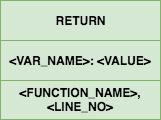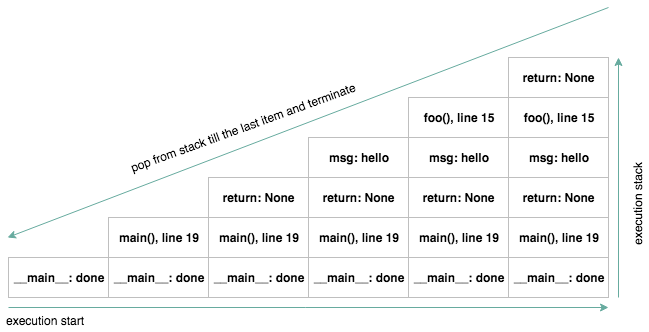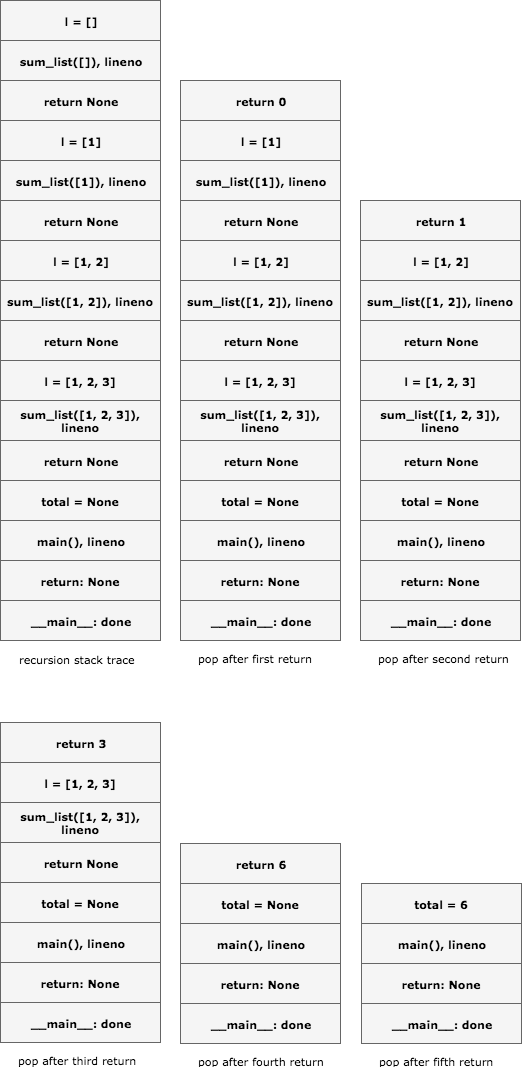# Python: function call, run time stack and recursion

Consider the code snippet below which has two functions `foo` and `main`. A special variable `__name__` which is fundamentally set by the python interpreter before execution and its value is set to `__main__` when executing as a main program.

In python, if a function does not end with a return statement or has a return statement without any expression, the special value `None` is returned.

``````#!/usr/bin/python

def foo(msg):
print '{} foo'.format(msg)

def main():
msg = 'hello'
foo(msg)

if __name__ == '__main__':
main()

# end
``````

In order to determine the output of above code we need to know in which order python executes lines of code. Of course we can run it and get the output but that’s not the point here.

When python program is executed using interpreter all the code at level 0 indentation is set to be run. In case above `main()` method will be executed. That’s the starting point of program execution and execution progress is kept in run time stack.

# What is run time stack? #

This is the simple run time stack in which call frames are added on to stack and they are popped one by one until the it reaches the last frame in the stack and then execution is terminated.This is the run time stack for our simple example program.Each block in the stack is called call frame aka activation records. They are stored in the memory to keep track of function call in progress and allocated memory is released when function returns.

Call frame is added on top of stack when function is called and removed when function returns. It contains the function name that was called and where to pick up from(line number) when function returns.

Parameters and local variables defined inside the function are also push onto stack and popped when function returns.When calling a function with parameters, the parameters becomes local variable on the stack that are initialized with actual parameter value.

So even if two functions have same local variable name they are different as they appear differently on to the stack. Same function call from different places possibly have different value for the same variables. Understanding this concept is important to understand how recursion works.

Below is the simple program to add numbers from a list using recursion.

``````#!/usr/bin/python

def sum_list(l):
if not l:
return 0
return l + sum_list(l[1:])

def main(l):
total = sum_list(l)
print total

if __name__ == '__main__':
main([1, 2, 3])

# end
``````

Below is the stack trace of above program for recursively getting sum of numbers in list.Understanding recursion under the hood can help us see its working mechanism which is not a magic. And writing recursion function required a base case to determine when to terminate. Without a base case it will stuck in loop and terminate with error.

### Python Generators: yield

In this post i will try to explain the basics of python generator. And will be writing another post to cover advance use and some debugging techniques. In python, generator is part of functional programming. A generator is an object that... Continue →